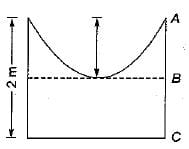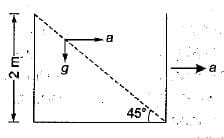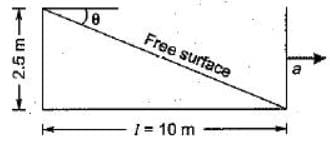Test: Liquids in Relative Equilibrium

# Test: Liquids in Relative Equilibrium

Test Description

## 9 Questions MCQ Test GATE Civil Engineering (CE) 2023 Mock Test Series | Test: Liquids in Relative Equilibrium

Test: Liquids in Relative Equilibrium for Civil Engineering (CE) 2022 is part of GATE Civil Engineering (CE) 2023 Mock Test Series preparation. The Test: Liquids in Relative Equilibrium questions and answers have been prepared according to the Civil Engineering (CE) exam syllabus.The Test: Liquids in Relative Equilibrium MCQs are made for Civil Engineering (CE) 2022 Exam. Find important definitions, questions, notes, meanings, examples, exercises, MCQs and online tests for Test: Liquids in Relative Equilibrium below.
Solutions of Test: Liquids in Relative Equilibrium questions in English are available as part of our GATE Civil Engineering (CE) 2023 Mock Test Series for Civil Engineering (CE) & Test: Liquids in Relative Equilibrium solutions in Hindi for GATE Civil Engineering (CE) 2023 Mock Test Series course. Download more important topics, notes, lectures and mock test series for Civil Engineering (CE) Exam by signing up for free. Attempt Test: Liquids in Relative Equilibrium | 9 questions in 30 minutes | Mock test for Civil Engineering (CE) preparation | Free important questions MCQ to study GATE Civil Engineering (CE) 2023 Mock Test Series for Civil Engineering (CE) Exam | Download free PDF with solutions
 1 Crore+ students have signed up on EduRev. Have you?
Test: Liquids in Relative Equilibrium - Question 1

### An oil tanker is partially filled and is then moved in translation at uniform acceleration. The free surface of oil then

Detailed Solution for Test: Liquids in Relative Equilibrium - Question 1

In this case the surface of constant pressure is a downward sloping plane surface. The free surface of the liquid is a surface of cohstant pressure, because the pressure at the free surface is equal to atmospheric pressure which is constant. As such under the effect of the horizontal acceleration the free surface of the liquid will become a downward sloping inclined plane, with the liquid rising at the back end and the liquid falling at the front end.

Test: Liquids in Relative Equilibrium - Question 2

### The free surface of a liquid partially filled in a cylindrical container when subjected to uniform rotation assumes a shape which can be represented by

Detailed Solution for Test: Liquids in Relative Equilibrium - Question 2

The shape of the free surface is paraboloid.

Test: Liquids in Relative Equilibrium - Question 3

### A closed tank containing water to a depth of 1.5 m falls vertically with an acceleration of 2 m/s2. What is the pressure at the bottom of the tank?

Detailed Solution for Test: Liquids in Relative Equilibrium - Question 3

Pressure at bottom of tank
= p (g - a) h = 1000 x (9.81 - 2) x 1.5
= 11.7 kN/m2

Test: Liquids in Relative Equilibrium - Question 4

A cylindrical vessel with a constant plane area of 1 m2 is rotated about its vertical axis such that the liquid inside the vessel is about to spill. If the height of the vessel is 2 m and the height of the paraboloid is 1 m, then the volume (in m3) of the liquid in the vessel will be

Detailed Solution for Test: Liquids in Relative Equilibrium - Question 4height of paraboloid = Z = 1 m = r2w2/2g
VoI. of water = VAB + VBC
VAB = Volume of paraboloid
= 1/2 volume of circumscribing cylinder
VBC = Cross- sectional area x 1
1/2 x 1 x 1 = 0.5 m3
Total volume of water = 1.5 m3

Test: Liquids in Relative Equilibrium - Question 5

A right circular cylinder, open at the top is filled with liquid, sp. gr. 1.2 and rotated about its vertical axis at such speed that half the liquid spills out. The pressure at the centre of the bottom is

Detailed Solution for Test: Liquids in Relative Equilibrium - Question 5

After half the water has spilled out the vertex of the paraboloid will touch the bottom of cylinder thus pressure will be zero.

Test: Liquids in Relative Equilibrium - Question 6

An open rectangular wagon 5 m long partly filled with water is subjected to an acceleration of 1.96 m/s2. For bn spilling of water the level below the open top upto which water may be filled is

Test: Liquids in Relative Equilibrium - Question 7

When a cylindrical vessel containing liquid is revolved about its vertical axis at a constant angular velocity, the pressure

Detailed Solution for Test: Liquids in Relative Equilibrium - Question 7

Variation in pressure in horizontal direction in a rotating cylindrical vessel containing fluid is given by,
∂P/∂r = pw2r

Test: Liquids in Relative Equilibrium - Question 8

An open cubical tank of 2 m side is filled with water. If the tank is accelerated horizontally with an acceleration such that half of the water spills out, then the acceleration is equal to

Detailed Solution for Test: Liquids in Relative Equilibrium - Question 8⇒ tan 45º = a/g ⇒  a = g

Test: Liquids in Relative Equilibrium - Question 9

A 3 m wide, 2.5 m deep, 10 m long tank, open at the top, has oil standing to 1 m depth. The maximum horizontal acceleration that can be given to the tank without spilling the oil will nearly be

Detailed Solution for Test: Liquids in Relative Equilibrium - Question 9

In the case of maximum acceleration, the free surface will be such that one end will have 2.5m depth and the other will have zero depth. Equating volume contained by free surface to earlier volume;
l x 2.5/2 x 3 = 3 x 10 x 1
I = 20/2.5 = 8m
tanθ = 2.5/8 = a/g
a = 0.31g## GATE Civil Engineering (CE) 2023 Mock Test Series

25 docs|282 tests
 Use Code STAYHOME200 and get INR 200 additional OFF Use Coupon Code
Information about Test: Liquids in Relative Equilibrium Page
In this test you can find the Exam questions for Test: Liquids in Relative Equilibrium solved & explained in the simplest way possible. Besides giving Questions and answers for Test: Liquids in Relative Equilibrium, EduRev gives you an ample number of Online tests for practice

## GATE Civil Engineering (CE) 2023 Mock Test Series

25 docs|282 tests